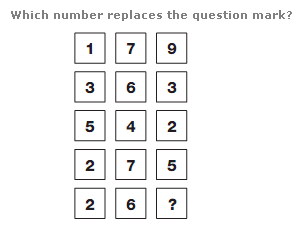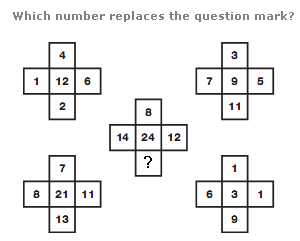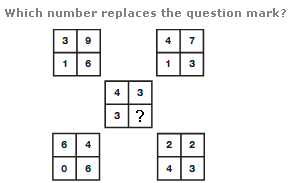# Puzzles - Number puzzles

Exercise : Number puzzles
37.:
7
Explanation
:
Reading each row of the diagram as a series of 3 digit numbers, the centre 3 digit number equals the sum of the top 2 numbers, and the sum of the lower 2 numbers.

38.:
22
Explanation
:
Add together values in corresponding positions of the top two crosses, and put the results in the lower left cross. Calculate the difference between values in corresponding positions of the top two crosses, and put the results in the lower right cross. Finally, add together the values in corresponding positions of the lower two crosses to give the values in the central cross.

39.